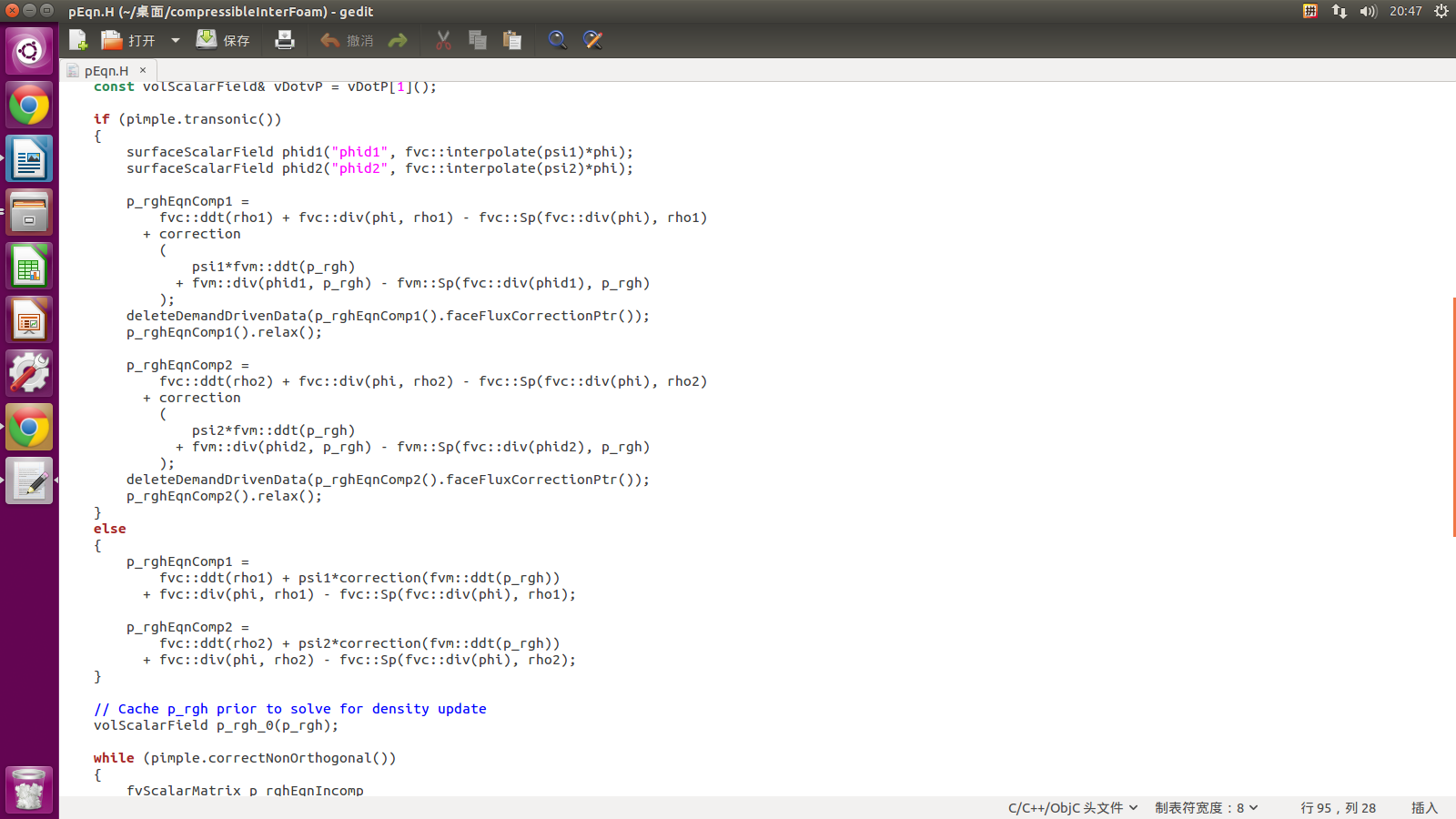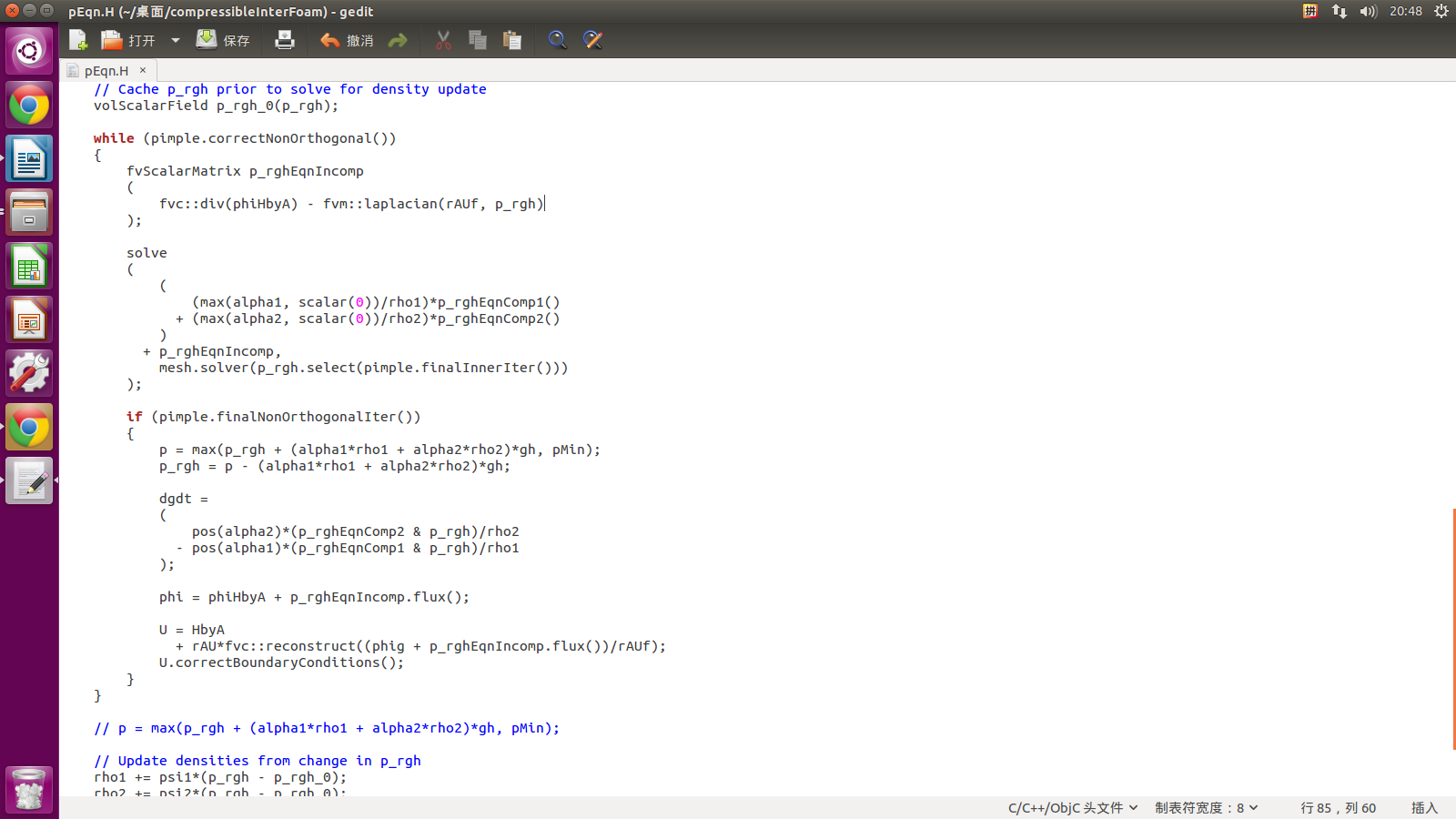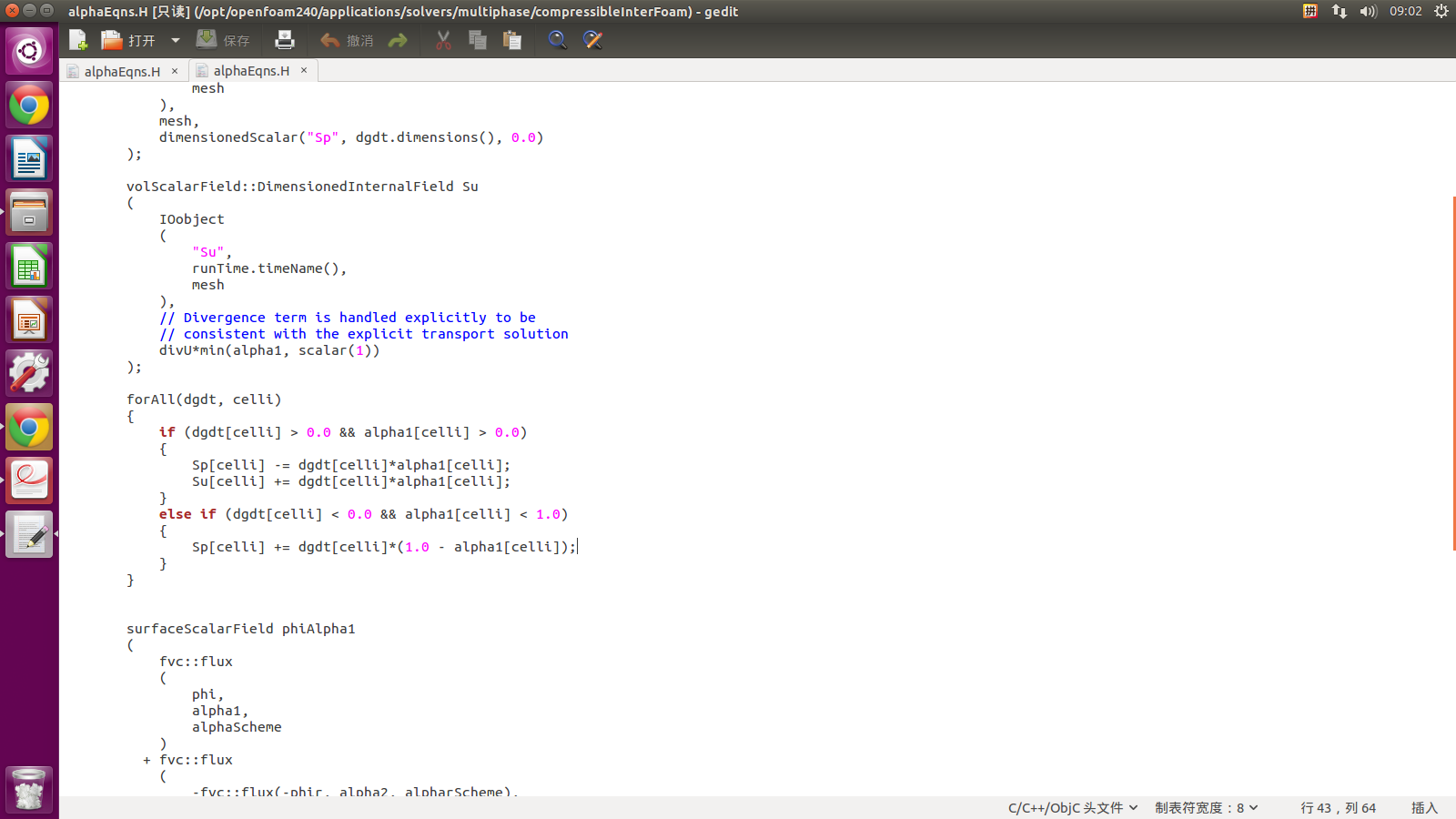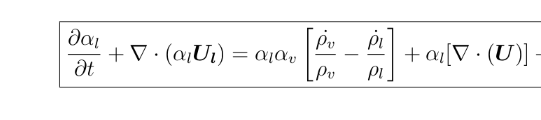# compressibleInterFoam 压力方程有懂的吗？

•• hi，

我刚看了一下，在之前发布的twoPhaseeulerfoam解析里面我没写压力方程。不过我这里有一个全面的，目前还没整理为网页的形式。需要很多时间整理，类似的帖子是：求解器中的dgdt在alpha方程是什么意思？

如果不着急我会在1个月内把interFoam和twoPhaseEulerFoam解析放出来一个，compressibleInterFoam只不过添加了压缩项。

或许，你可以更具体的说哪一行代码有问题？

• 第一张图片里面p_rghEqn1是怎么推导的呢？可以先给解释一下这部分吗

• @mark

这个方程推导还是非常费事的，这里可能说不太清楚。我刚刚放出了interFoam不可压缩部分的方程推导。过几天添加interFoam代码部分，然后谈价compressibleInterFoam的方程，以及代码。

• @李东岳 dgdt在alpha方程及p_rgh的意思及作用是？

• @mark

dgdt表示的是可压缩性。比如可压缩相方程为：
\begin{equation}
\frac{\partial \alpha_\phi \rho_\phi}{\partial t}+\nabla \cdot (\alpha_\phi \rho_\phi \mathbf{U}_\phi)=0
\end{equation}
经过大量的推导，dgdt项就是
\begin{equation}
dgdt=\frac{1}{\rho_2}\frac{\mathrm{D}\rho_1}{\mathrm{D}t}-\frac{1}{\rho_1}\frac{\mathrm{D}\rho_2}{\mathrm{D}t}
\end{equation}
其中省略了大量的推导步骤。不过最终的dgdt如此。

p_rghEqn1更麻烦了，基本解释不了在这里....

• @李东岳可以帮我解释一下forAll循环里面的代码吗？谢谢！

•• @mark Hi,

等我有空把文档写完，那个暂时一句俩句说不清楚。